# How to Get Length of Array in Excel VBA?

• Last Updated : 22 Nov, 2021

We use UBound and LBound functions to get the length of an Array in Excel VBA. In this article, we will discuss them in detail.

Syntax: UBound() function

`UBound (arrayname, [ dimension ])`

Parameters:

• arrayname: required. Array variable name
• dimension: optional

Returns: Return upper limit of an array dimension.

Syntax: LBound() Function

`LBound (arrayname, [ dimension ])`

Parameters:

• arrayname : required. Array variable name
• dimension : optional

Returns: Return lower limit of an array dimension

Sample Data: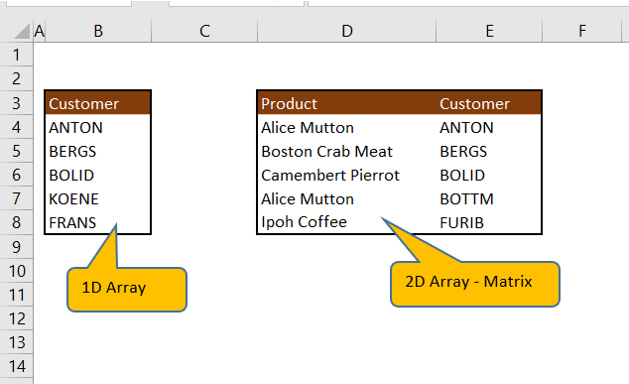### VBA Code to get the length of Array (one-dimensional array):

Declare Variables:

Declaring a customer array with the size of 10.

```Sub oneDimArrayLength()
' Array variable Declaration
Dim customer (1 To 10) As String```

Assign values to array elements

```customer(1) = "ANTON"
customer(2) = "BERGS"
customer(3) = "BOLID"
customer(4) = "KOENE"
customer(5) = "FRANS"```

Use UBound function to get the size of an array and Message box to display the result

```'Message box to popup length of 1D array
MsgBox "Array has " & UBound(customer) & " element(s)."

End Sub```

To Run VBA Code

Press Alt+F8 to popup macro window.  Select ” oneDimArrayLength” and Click Run button.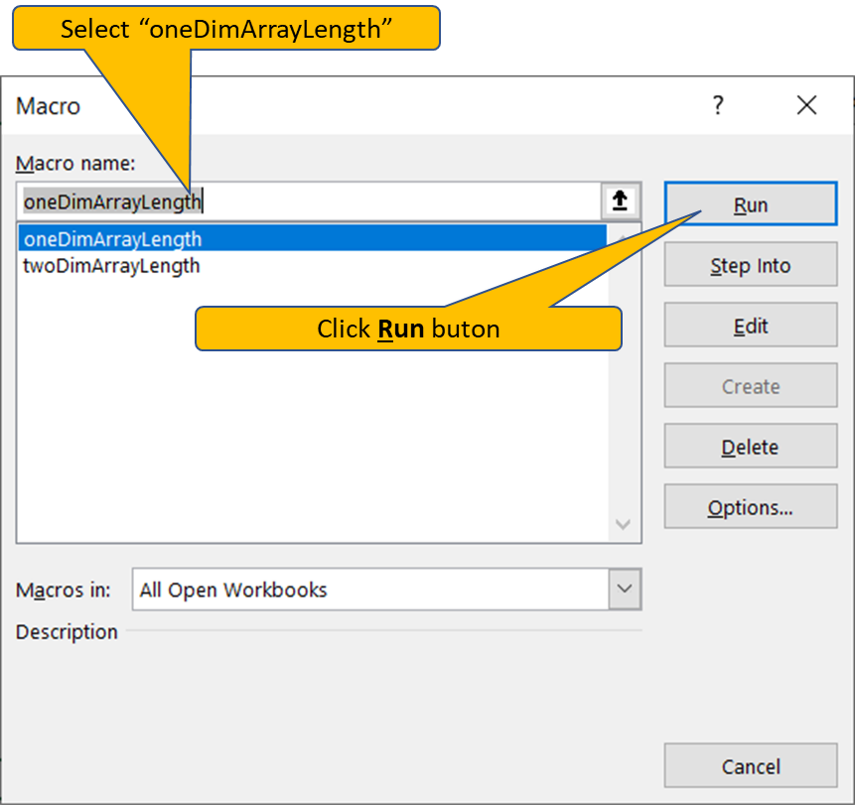Output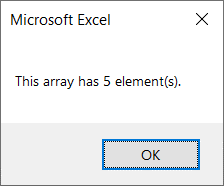VBA Code to get the length of Array (multi-dimensional array)

Declaring variables:

Declaring ProdAndCustomer multi-dimensional array size of 10 rows and 2 columns

```Sub twoDimArrayLength()

' Array variable Declaration
Dim ProdAndCustomer(1 To 10, 1 To 2) As String, noOfRow As Integer, noOfCol As Integer, noOfElements As Integer```

Assign values to array elements

```ProdAndCustomer(1, 1) = "Alice Mutton"
ProdAndCustomer(2, 1) = "Boston Crab Meat"
ProdAndCustomer(3, 1) = "Camembert Pierrot"
ProdAndCustomer(4, 1) = "Alice Mutton"
ProdAndCustomer(5, 1) = "Ipoh Coffee"

ProdAndCustomer(1, 2) = "ANTON"
ProdAndCustomer(2, 2) = "BERGS"
ProdAndCustomer(3, 2) = "BOLID"
ProdAndCustomer(4, 2) = "BOTTM"
ProdAndCustomer(5, 2) = "FURIB"```

Compute Number of Rows, Number of Columns using UBound and LBound function.  Multiply by noOfRow and noOfCol variable to get Number of elements in multi-dimensional array.

```noOfRow = UBound(ProdAndCustomer, 1) - LBound(ProdAndCustomer, 1) + 1
noOfCol = UBound(ProdAndCustomer, 2) - LBound(ProdAndCustomer, 2) + 1
noOfElements = noOfRow * noOfCol```

Message box to popup result

```'Message box to popup length of 1D array
MsgBox "Array has " & noOfElements & " element(s)."

End Sub```

To Run VBA Code

Press Alt+F8 to popup macro window.  Select ” twoDimArrayLength” and Click Run button.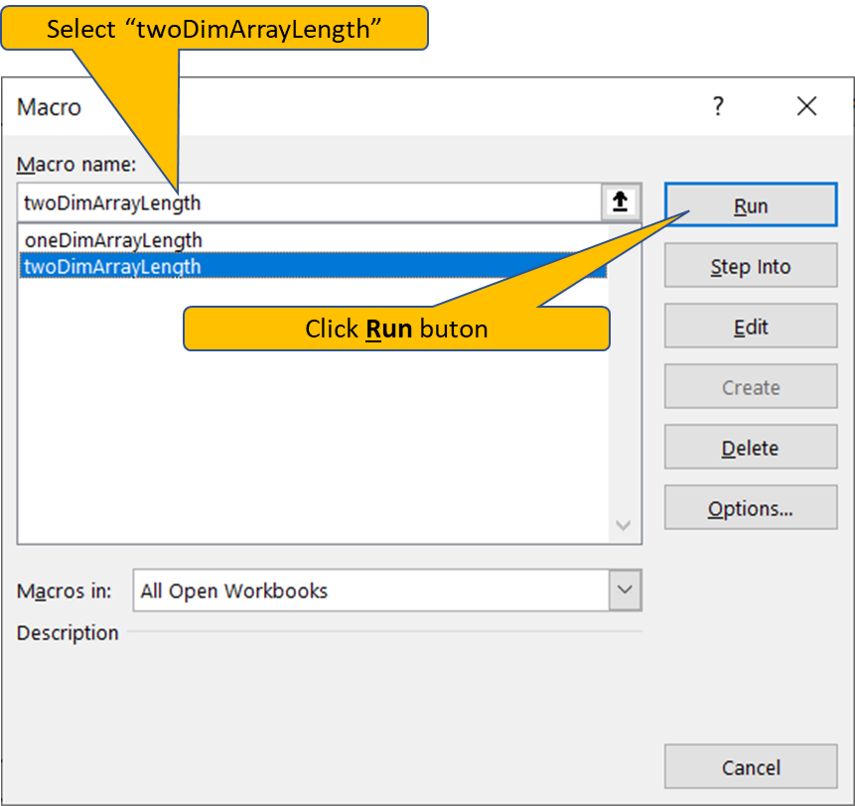Output: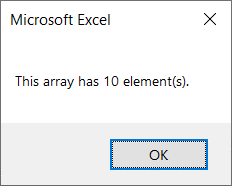My Personal Notes arrow_drop_up